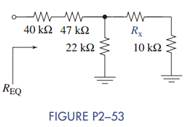Chat Now

# Select the value of Rx in Figure P2−53 so that R EQ = 100 kΩ.

### Select the value of Rx in Figure P2−53 so that R EQ = 100 kΩ.

Select the value of Rx in Figure P2−53 so that REQ = 100 kΩ.manish jayant 28-Jul-2020Get solution